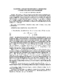## Modified Streamline Diffusion Schemes for Convection-Diffusion Problems##### Files
CS-TR-3835.ps(849.06 KB)
We consider the design of robust and accurate finite element approximation methods for solving convection--diffusion problems. We develop some two--parameter streamline diffusion schemes with piecewise bilinear (or linear) trial functions and show that these schemes satisfy the necessary conditions for $L^{2}$-uniform convergence of order greater than $1/2$ introduced by Stynes and Tobiska. For smooth problems, the schemes satisfy error bounds of the form $O(h)|u|_{2}$ in an energy norm. In addition, extensive numerical experiments show that they effectively reproduce boundary layers and internal layers caused by discontinuities on relatively coarse grids, without any requirements on alignment of flow and grid. (Also cross-referenced as UMIACS-TR-97-71)